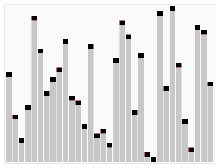# 1 快速排序//
//  QuickSort.swift
//  QuickSort
//
//  CSDN:http://blog.csdn.net/y550918116j
//  GitHub:https://github.com/937447974/Blog
//
//  Created by yangjun on 15/11/16.
//  Copyright © 2015年 阳君. All rights reserved.
//

import Foundation

/// 快速排序
class QuickSort: NSObject {

/// 数组
var list = Array<Int>()

}

## 1.1 分治过程

### 1.1.1 分解

list[first..location-1]的每一个元素都小于list[location]，而list[location]也小于等于[location+1..end]中的每个元素。即

list[first..location-1] <= list[location] <=list[location+1..end]

## 1.2.1 核心代码

// MARK: - 快速排序
func quickSort() {
self.quickSort(0, end: self.list.count-1)
}

// MARK: 分解
private func quickSort(first:Int, end:Int) {
if first < end {
// 获取中位点
let location = self.partition(first, end: end)
self.quickSort(first, end: location-1)
self.quickSort(location+1, end: end)
}
}

// MARK: 排序核心算法
private func partition(first:Int, end:Int) ->Int {
var index = first - 1
for(var i = first; i < end; i++) {
if self.list[i] < self.list[end] {
index++
self.exchange(i, index)
}
}
index++
self.exchange(index, end)
return index
}

// MARK: 交换数据
private func exchange(p:Int, _ r:Int){
if p == r {
return
}
let temp = self.list[p]
self.list[p] = self.list[r]
self.list[r] = temp
}

## 1.2.1 核心代码解说

indexfirst、iend
27814

index在-1位，i在首尾。i会从first移动到end。当i移动到0位时，2<4。此时index移动到0位。

index、firstiend
27814

i继续移动，移动到3位时，1<4。index移动到1位，交换index和i位置的数据，也就是交换1和7。

firstindexiend
21874

firstindexiend
21478

# 2 快速排序的性能

## 2.1 最坏情况

T(n) = T(n-1) + o(n)

## 2.2 最好情况划分

T(n) = 2T(n/2) + o(n)

# 3 随机快速排序

list[first..end-1] <= list[end] 或 list[first..end-1] >= list[end]

// MARK: - 随机快速排序
func radomQuickSort() {
self.radomQuickSort(0, end: self.list.count-1)
}

// MARK: 开始随机排序
private func radomQuickSort(first:Int, end:Int) {
if first < end {
// 获取中位点
let location = self.radomPartition(first, end: end)
self.radomQuickSort(first, end: location-1)
self.radomQuickSort(location+1, end: end)
}
}

// MARK: 获取划分的位置
private func radomPartition(first:Int, end:Int) ->Int {
// 随机一个数放到尾部，主要防止遇到最坏排序情况，即数组为倒序
// 获取first-end的随机数
let random = arc4random_uniform(UInt32(end-first))
// 或 random = Int(arc4random()) % (end-first)
let index = Int(random) + first
self.exchange(index, end);
return self.partition(first, end: end)
}

# 4 多线程快速排序

// MARK: - 多线程快速排序
func threadQuickSort() {
self.threadQuickSort(0, end: self.list.count-1)
}

// MARK: 开始多线程快速排序
private func threadQuickSort(first:Int, end:Int) {
// 在随机排序的基础上启用多线程排序
if first < end {
// 获取中位点
let location = self.radomPartition(first, end: end)
// 分到多个线程排序，并发
let queue = dispatch_get_global_queue(DISPATCH_QUEUE_PRIORITY_DEFAULT, 0)
dispatch_async(queue, { () -> Void in
self.threadQuickSort(first, end: location-1)
})
dispatch_async(queue, { () -> Void in
self.threadQuickSort(location+1, end: end)
})
}
}

# 5 测试

## 5.1 测试代码

// 快速排序测试
let quickSort = QuickSort()
quickSort.list.append(0)
for(var i = 10; i > 0; i--){
quickSort.list.append(i)
}
quickSort.quickSort()
print("快速排序:\(quickSort.list)")

// 速度测试，使用最坏情况测试，也就是原数组为倒序
let count = 10000 // 要排序的元素个数
var source = Array<Int>()
for(var i = count; i > 0; i--){
source.append(i)
}
print("快排速度测试,总数：\(count)")

// 快速排序
var date = NSDate()
quickSort.list = source
quickSort.quickSort()
print("快速排序耗时:\(-date.timeIntervalSinceNow)")

// 随机快速排序
date = NSDate()
quickSort.list = source
quickSort.radomQuickSort()
print("随机快速排序耗时:\(-date.timeIntervalSinceDate(NSDate()))")

// 多线程快速排序
date = NSDate()
quickSort.list = source
quickSort.threadQuickSort()
print("多线程快速排序耗时:\(-date.timeIntervalSinceNow)")

## 5.2 结果

快速排序:[0, 1, 2, 3, 4, 5, 6, 7, 8, 9, 10]

# 其他

## 源代码

https://github.com/937447974/Algorithms

IOS多线程

## 文档修改记录

2015-11-16算法项目完成、博文完成

## 版权所有

03-31577403-041万+
03-133012
08-151103
09-152万+
12-181337
10-053973
09-124万+
03-151282
12-229861
10-2067
11-212432
12-20430
04-079607
05-25517
10-272672
07-173049
©️2020 CSDN 皮肤主题: 编程工作室 设计师:CSDN官方博客